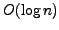# On the Computational Power of Threshold Circuits with Sparse Activity

K. Uchizawa, R. Douglas, and W. Maass

### Abstract:

Circuits composed of threshold gates (McCulloch-Pitts neurons, or perceptrons) are simplified models of neural circuits with the advantage that they are theoretically more tractable than their biological counterparts. However, when such threshold circuits are designed to perform a specific computational task they usually differ in one important respect from computations in the brain: they require very high activity. On average every second threshold gate fires (sets a ``1'' as output) during a computation. By contrast, the activity of neurons in the brain is much more sparse, with only about 1% of neurons firing. This mismatch between threshold and neuronal circuits is due to the particular complexity measures (circuit size and circuit depth) that have been minimized in previous threshold circuit constructions. In this article we investigate a new complexity measure for threshold circuits, energy complexity, whose minimization yields computations with sparse activity. We prove that all computations by threshold circuits of polynomial size with entropycan be restructured so that their energy complexity is reduced to a level near the entropy of circuit states. This entropy of circuit states is a novel circuit complexity measure, which is of interest not only in the context of threshold circuits, but for circuit complexity in general. As an example of how this measure can be applied we show that any polynomial size threshold circuit with entropycan be simulated by a polynomial size threshold circuit of depth 3. Our results demonstrate that the structure of circuits which result from a minimization of their energy complexity is quite different from the structure which results from a minimization of previously considered complexity measures, and potentially closer to the structure of neural circuits in the nervous system. In particular, different pathways are activated in these circuits for different classes of inputs. This article shows that such circuits with sparse activity have a surprisingly large computational power.

Reference: K. Uchizawa, R. Douglas, and W. Maass. On the computational power of threshold circuits with sparse activity. Neural Computation, 18(12):2994-3008, 2006.